Fractions No Prep Introduction to Fractions Workbook
Fractions No Prep Workbook - Not Boring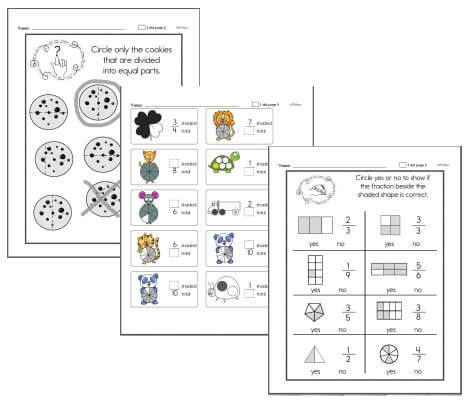Kindergarten - Introduction to Fractions Workbook First Grade - Introduction to Fractions Workbook Second Grade - Introduction to Fractions Workbook

Fraction I Have... Who Has...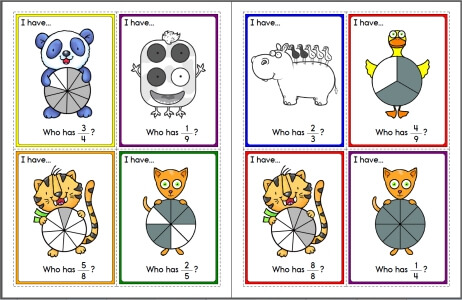Fraction I Have... Who Has... [4 per page - animal pictures]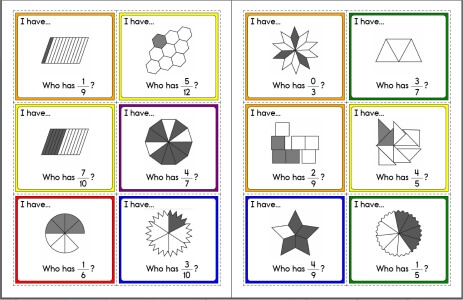Fraction I Have... Who Has... [6 per page]

Fraction Charts for Bulletin Board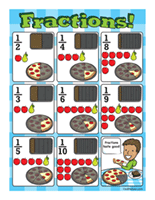Fractions! (8.5 x 11)Fractions! (17 x 22)

Fraction Number Lines

Runaway Math Puzzles with FractionsRunaway Math Puzzles with Fractions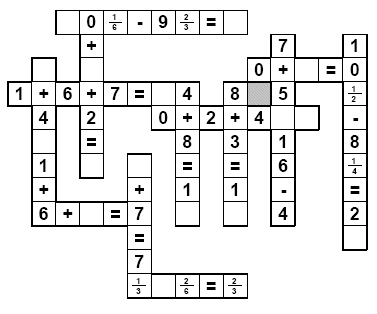Fractions (Grades K-2)
One half - circle the shape
One half - color
One third - circle the shape
One third - color
One fourth - circle the shape
One fourth - color
What fraction does the colored part show? Circle the fraction
Color one part
Draw lines to show the fraction
Parts of a group
More parts of a group
Symmetry
Fractions and pizza

Fractions Review
Fractions Review
Fractions Review (larger review and includes pizza word problems)

Understand Fractions (Grades 2-3)
Write a fraction to show how much of the shape is shaded
Write a fraction to show how much of the shape is not shaded
Draw a picture to show the fraction
Fraction Comparison (with shaded graphics)
Same numerators
Same denominators
Either the same numerators or the same denominators
Fraction Comparison (with graphics, but not shaded in)
Same numerators
Same denominators
Fill in the missing number to make two equivalent fractions
Fill in one number: Larger graphics with fractions shown
Fill in one number: Smaller graphics with only boxes
Fill in two number: Larger graphics with fractions shown
Fill in two number: Smaller graphics with only boxes
Mixed Numbers and Improper Fractions
Multiple choice: Circle the correct improper fraction for the parts that are shaded
Multiple choice: Circle the correct mixed number for the parts that are shaded
Write an improper fraction for the parts that are shaded
Write a mixed number for the parts that are shaded
Problem Solving
Single step
Two steps
Mixed Review
Understand Fractions Mixed Review

Fractions Math Center - Write the Fraction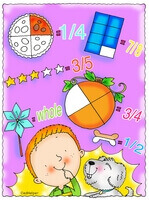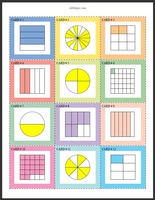Write the fraction - numerator is always 1 (Grades 2-4) Write the fraction (Grades 3-6) Write the fraction - a blank on student page (student will need to know to draw line and where to put numerator and denominator) (Grades 3-6)

Matching - Picture of Fractions and Fractions - Math Learning Center

Matching - Picture of Fractions, Fractions, and Decimals - Math Learning Center

Ordering Numbers Math Center - Fractions

Add and Subtract Like Fractions (Grade 3)
Fraction comparison
Order fractions from least to greatest
Order fractions from greatest to least
Rewrite each improper fraction as a mixed number
Add fractions
Add fractions: Find the value of y
Subtract fractions
Subtract fractions: Find the value of y
Add and subtract fractions
Add and subtract fractions: Find the value of y
Add and Subtract Like Fractions Mixed Review

Number Theory
Divisibility: circle all of the numbers that are divisible by the number
Divisibility: complete the table
Prime or composite
Use the clue to fill in the missing digit
Circle all of the numbers that are multiples of a number
List 3 multiples of each number
Fill in the missing multiple
List all of the factors
Prime factorization
Greatest common factor
Least common multiple
Open-ended number theory questions
Number theory review printable

Understand Fractions (Grades 4-5)
Circle the equivalent fraction
Write two equivalent fractions
Is each fraction in simplest form?
Write each fraction in simplest form
Equivalent fractions: fill in the missing number (easier)
Equivalent fractions: fill in the missing number
Comparing fractions
Ordering fractions (from least to greatest)
Ordering fractions (from greatest to least)
Part of a number
Problem solving
Circle the equivalent improper fraction
Circle the equivalent mixed number
Write each improper fraction as a mixed number
Write each mixed number as an improper fraction
Open-ended fraction problems
Understand Fractions Mixed Review

Add and Subtract Fractions
Add Fractions with Like Denominators
Add fractions with like denominators (horizontal)
Add fractions with like denominators (vertical)
Add fractions with like denominators: find the value of y
Subtract Fractions with Like Denominators
Subtract fractions with like denominators (horizontal)
Subtract fractions with like denominators (vertical)
Subtract fractions with like denominators: find the value of y
Add and Subtract Fractions with Like Denominators
Add and subtract fractions with like denominators (horizontal)
Add and subtract fractions with like denominators (vertical)
Add and subtract fractions with like denominators: find the value of y
Add and Subtract Fractions with Like Denominators (Mixed Numbers)
Add and subtract fractions with like denominators and mixed numbers (horizontal)
Add and subtract fractions with like denominators and mixed numbers (vertical)
Add and subtract fractions with like denominators and mixed numbers: find the value of y
Add Fractions with Unlike Denominators
Add fractions with unlike denominators (horizontal)
Add fractions with unlike denominators (vertical)
Add fractions with unlike denominators: find the value of y
Subtract Fractions with Unlike Denominators
Subtract fractions with unlike denominators (horizontal)
Subtract fractions with unlike denominators (vertical)
Subtract fractions with unlike denominators: find the value of y
Add and Subtract Fractions with Unlike Denominators
Add and subtract fractions with unlike denominators (horizontal)
Add and subtract fractions with unlike denominators (vertical)
Add and subtract fractions with unlike denominators: find the value of y
Add and Subtract Fractions with Unlike Denominators (Mixed Numbers)
Add and subtract fractions with unlike denominators and mixed numbers (horizontal)
Add and subtract fractions with unlike denominators and mixed numbers (vertical)
Add and subtract fractions with unlike denominators and mixed numbers: find the value of y
Problem Solving
Find parts of a number
Addition and subtraction of fractions
Addition and subtraction of fractions (mixed numbers)
Addition and subtraction of fractions (multi-step)
Addition and subtraction of fractions (more problems)
Review
Add and Subtract Fractions Mixed Review

Fraction Word Problems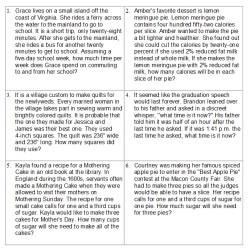Fraction Word Problems (3rd grade) Fraction Word Problems (4th grade) Fraction Word Problems (5th grade) Fraction Word Problems (6th grade)

Understanding Decimals
Tenths
Write each as a decimal (given: graphics, fractions, and word form)
Given graphics, write the decimal (decimals greater than 1)
Write each as a decimal (decimals greater than 1)
Write each as a fraction

Tenths and Hundredths
Hundredths: Write each as a fraction and decimal
Hundredths: Write each as a decimal
Hundredths: Write each as a fraction
Tenths and hundredths: Write each as a decimal
Tenths and hundredths: Write each as a fraction
Hundredths: Write each as a decimal (graphics)
Tenths and hundredths greater than 1: Write each as a decimal
Tenths and hundredths greater than 1: Write each as a fraction
Tenths and hundredths: Write each as a decimal (mix: less than and greater than 1 decimals)
Tenths and hundredths: Write each as a fraction (mix: less than and greater than 1 decimals)
Tenths and hundredths: Compare decimals
Tenths and hundredths: Order decimals
Order decimals (from 6 to 8 numbers each)
Compare decimals and fractions
Order decimals and fractions
Order decimals and fractions (from 7 to 11 numbers each)
Compare decimals and fractions (with numbers greater than 1)
Order decimals and fractions (with numbers greater than 1)
Tenths and Hundredths Mixed Review

Thousandths
Thousandths: Write as a decimal
Thousandths: Write as a fraction
Thousandths: Write as a decimal (decimals greater than 1)
Thousandths: Write as a fraction (decimals greater than 1)

Tenths, Hundredths, and Thousandths
Write as a decimal
Write as a fraction
Compare decimals
Ordering decimals
Compare decimals (including fractions)
Ordering decimals (including fractions)

Rounding Decimals
Rounding: Round to the nearest tenth
Rounding: Round to the nearest hundredth
Rounding: Round to the place of the underlined digit

Review
Mixed Review

Fractions
Equivalent fractions
Simplify fractions
Comparing fractions
Ordering fractions
Write improper fractions as mixed numbers
Write mixed numbers as improper fractions
Fill in the missing number
Adding and subtracting like denominators
Multiplying fractions by whole numbers
Multiplying fractions
Multiplying mixed numbers
Dividing fractions
Dividing mixed numbers
Adding and subtracting unlike denominators (whole numbers)
Adding and subtracting unlike denominators
Adding and subtracting unlike denominators (mixed numbers)
Solving addition and subtraction equations (algebra)
Solving multiplication and division equations (algebra)
Open-ended fraction problems (easier)
Open-ended fraction problems
Fractions review printable

Fractions - Word Problems (middle school and high school level problems)
General Fractions Word Problems
Biology
Geology
Physics
Chemistry
Sports
Fractions Word Problems Mixed Review

Repeating Decimals
Write a fraction as a decimal
Terminating decimals only (denominators of 2-20)
Repeating decimals only (denominators of 2-20)
Terminating and repeating decimals (denominators of 2-20)
Write a fraction (all in lowest terms) as a decimal (easier fractions)
Write a fraction as a decimal (larger denominators)
Write a fraction (all in lowest terms) as a decimal
Write a fraction as a decimal
Write a fraction as a decimal (all in lowest terms)
Write a decimal as a fraction
Write terminating decimals as fractions
Write repeating decimals as fractions (includes instructions)
Write repeating decimals as fractions
Ordering
Order 3 numbers
Order 7 numbers
Order 7 numbers (includes negatives)
Repeating Decimals Mixed Review

Simplifying Algebraic Fractions (Some Polynomials)
Reducing Algebraic Fractions to Lowest Terms (Warm Up)
Reducing Algebraic Fractions to Lowest Terms (A Little More Difficult)
Reducing Algebraic Fractions to Lowest Terms (Different Variables)
Multiplying Algebraic Fractions
Adding Algebraic Fractions
Subtracting Algebraic Fractions
Adding and Subtracting Algebraic Fractions
Adding Algebraic Fractions (More Difficult)
Subtracting Algebraic Fractions (More Difficult)
Adding and Subtracting Algebraic Fractions (More Difficult)
Final Review

Fraction Number Puzzles
* The following worksheets are for edHelper.com subscribers.
A subscription to edHelper includes access to the math, spelling, and vocabulary subscription areas. Sign up for the subscriber materials.
Writing Tenths Fractions and Decimals
Writing Hundredths Fractions and Decimals
Reduce Improper Fractions to Lowest Terms
Reduce to Lowest Terms
Write Fraction as a Decimal (10 and 100 Denominators)
Write Fraction as a Decimal
Write Mixed Number (includes Number Words) as a Decimal
Write Decimal as a Fraction
Fill in the Missing Numerator or Denominator
Addition (Like Denominators)
Subtraction (Like Denominators)
Addition (Like Denominators - Reduce to Lowest Terms)
Subtraction (Like Denominators- Reduce to Lowest Terms)
Addition and Subtraction (Like Denominators)
Addition (Different Denominators)
Subtraction (Different Denominators)
Addition and Subtraction
Multiplication
Multiplication (Mixed Fractions)
Division
Division (Mixed Fractions)
Multiplication and Division
Addition,Subtraction,Multiplication,Division

Learn about Fractions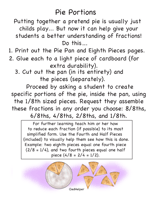Pie Portions

Have a suggestion or would like to leave feedback?
Leave your suggestions or comments about edHelper!